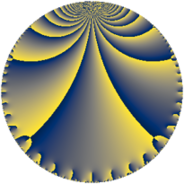# Properties

 Label 784.4.f.eLevel $784$ Weight $4$ Character orbit 784.f Analytic conductor $46.257$ Analytic rank $0$ Dimension $4$ CM discriminant -4 Inner twists $4$

# Related objects

## Newspace parameters

 Level: $$N$$ $$=$$ $$784 = 2^{4} \cdot 7^{2}$$ Weight: $$k$$ $$=$$ $$4$$ Character orbit: $$[\chi]$$ $$=$$ 784.f (of order $$2$$, degree $$1$$, minimal)

## Newform invariants

 Self dual: no Analytic conductor: $$46.2574974445$$ Analytic rank: $$0$$ Dimension: $$4$$ Coefficient field: 4.0.2048.2 Defining polynomial: $$x^{4} + 4 x^{2} + 2$$ Coefficient ring: $$\Z[a_1, \ldots, a_{13}]$$ Coefficient ring index: $$7^{2}$$ Twist minimal: yes Sato-Tate group: $\mathrm{U}(1)[D_{2}]$

## $q$-expansion

Coefficients of the $$q$$-expansion are expressed in terms of a basis $$1,\beta_1,\beta_2,\beta_3$$ for the coefficient ring described below. We also show the integral $$q$$-expansion of the trace form.

 $$f(q)$$ $$=$$ $$q + ( -\beta_{1} - 5 \beta_{2} ) q^{5} -27 q^{9} +O(q^{10})$$ $$q + ( -\beta_{1} - 5 \beta_{2} ) q^{5} -27 q^{9} + ( 4 \beta_{1} + 21 \beta_{2} ) q^{13} + ( 6 \beta_{1} - 29 \beta_{2} ) q^{17} + ( -125 - 23 \beta_{3} ) q^{25} -11 \beta_{3} q^{29} -13 \beta_{3} q^{37} + ( -51 \beta_{1} + 83 \beta_{2} ) q^{41} + ( 27 \beta_{1} + 135 \beta_{2} ) q^{45} + 572 q^{53} + ( -152 \beta_{1} - 41 \beta_{2} ) q^{61} + ( 1048 + 97 \beta_{3} ) q^{65} + ( 163 \beta_{1} + 193 \beta_{2} ) q^{73} + 729 q^{81} + ( -1332 - 157 \beta_{3} ) q^{85} + ( -226 \beta_{1} + 157 \beta_{2} ) q^{89} + ( -217 \beta_{1} + 257 \beta_{2} ) q^{97} +O(q^{100})$$ $$\operatorname{Tr}(f)(q)$$ $$=$$ $$4q - 108q^{9} + O(q^{10})$$ $$4q - 108q^{9} - 500q^{25} + 2288q^{53} + 4192q^{65} + 2916q^{81} - 5328q^{85} + O(q^{100})$$

Basis of coefficient ring in terms of a root $$\nu$$ of $$x^{4} + 4 x^{2} + 2$$:

 $$\beta_{0}$$ $$=$$ $$1$$ $$\beta_{1}$$ $$=$$ $$\nu^{3}$$ $$\beta_{2}$$ $$=$$ $$2 \nu^{3} + 7 \nu$$ $$\beta_{3}$$ $$=$$ $$7 \nu^{2} + 14$$
 $$1$$ $$=$$ $$\beta_0$$ $$\nu$$ $$=$$ $$($$$$\beta_{2} - 2 \beta_{1}$$$$)/7$$ $$\nu^{2}$$ $$=$$ $$($$$$\beta_{3} - 14$$$$)/7$$ $$\nu^{3}$$ $$=$$ $$\beta_{1}$$

## Character values

We give the values of $$\chi$$ on generators for $$\left(\mathbb{Z}/784\mathbb{Z}\right)^\times$$.

 $$n$$ $$197$$ $$687$$ $$689$$ $$\chi(n)$$ $$1$$ $$-1$$ $$-1$$

## Embeddings

For each embedding $$\iota_m$$ of the coefficient field, the values $$\iota_m(a_n)$$ are shown below.

For more information on an embedded modular form you can click on its label.

Label $$\iota_m(\nu)$$ $$a_{2}$$ $$a_{3}$$ $$a_{4}$$ $$a_{5}$$ $$a_{6}$$ $$a_{7}$$ $$a_{8}$$ $$a_{9}$$ $$a_{10}$$
783.1
 0.765367i − 1.84776i 1.84776i − 0.765367i
0 0 0 21.8561i 0 0 0 −27.0000 0
783.2 0 0 0 4.72352i 0 0 0 −27.0000 0
783.3 0 0 0 4.72352i 0 0 0 −27.0000 0
783.4 0 0 0 21.8561i 0 0 0 −27.0000 0
 $$n$$: e.g. 2-40 or 990-1000 Significant digits: Format: Complex embeddings Normalized embeddings Satake parameters Satake angles

## Inner twists

Char Parity Ord Mult Type
1.a even 1 1 trivial
4.b odd 2 1 CM by $$\Q(\sqrt{-1})$$
7.b odd 2 1 inner
28.d even 2 1 inner

## Twists

By twisting character orbit
Char Parity Ord Mult Type Twist Min Dim
1.a even 1 1 trivial 784.4.f.e 4
4.b odd 2 1 CM 784.4.f.e 4
7.b odd 2 1 inner 784.4.f.e 4
28.d even 2 1 inner 784.4.f.e 4

By twisted newform orbit
Twist Min Dim Char Parity Ord Mult Type
784.4.f.e 4 1.a even 1 1 trivial
784.4.f.e 4 4.b odd 2 1 CM
784.4.f.e 4 7.b odd 2 1 inner
784.4.f.e 4 28.d even 2 1 inner

## Hecke kernels

This newform subspace can be constructed as the kernel of the linear operator $$T_{3}$$ acting on $$S_{4}^{\mathrm{new}}(784, [\chi])$$.

## Hecke characteristic polynomials

$p$ $F_p(T)$
$2$ $$T^{4}$$
$3$ $$T^{4}$$
$5$ $$10658 + 500 T^{2} + T^{4}$$
$7$ $$T^{4}$$
$11$ $$T^{4}$$
$13$ $$2913698 + 8788 T^{2} + T^{4}$$
$17$ $$38596898 + 19652 T^{2} + T^{4}$$
$19$ $$T^{4}$$
$23$ $$T^{4}$$
$29$ $$( -11858 + T^{2} )^{2}$$
$31$ $$T^{4}$$
$37$ $$( -16562 + T^{2} )^{2}$$
$41$ $$18721512002 + 275684 T^{2} + T^{4}$$
$43$ $$T^{4}$$
$47$ $$T^{4}$$
$53$ $$( -572 + T )^{4}$$
$59$ $$T^{4}$$
$61$ $$11781432002 + 907924 T^{2} + T^{4}$$
$67$ $$T^{4}$$
$71$ $$T^{4}$$
$73$ $$580595793698 + 1556068 T^{2} + T^{4}$$
$79$ $$T^{4}$$
$83$ $$T^{4}$$
$89$ $$1399267092962 + 2819876 T^{2} + T^{4}$$
$97$ $$3254346436898 + 3650692 T^{2} + T^{4}$$